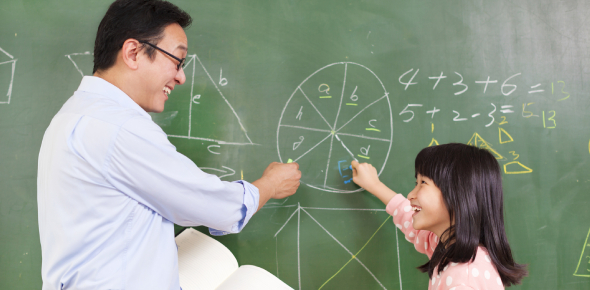# Basic Math Vocabulary Skill Test

15 Questions | Total Attempts: 1225Settings• 1.
A sum is_____________________________________________________
• A.

The difference of two numbers.

• B.

The total amount of two or more addends.

• C.

The greater number.

• D.

The smaller number

• E.

None of the above

• 2.
A difference is ____________________________________________
• A.

• B.

Found by comparing numbers or subtracting.

• C.

Found by multiplying.

• D.

Found by dividing.

• E.

Found using the lattice method.

• 3.
A  product is ______________________________________________
• A.

The result when two numbers are multiplied.

• B.

The result when two numbers are divided.

• C.

The result when two numbers are added.

• D.

The result when two numbers are subtracted.

• E.

The result when two numbers are compared.

• 4.
Parallel lines are always ____________________________________
• A.

Crossing each other.

• B.

Squiggly.

• C.

Part of a square.

• D.

The same distance apart.

• E.

Intersecting.

• 5.
A pound is a _____________________________________
• A.

Measurement tool.

• B.

A standard unit for measuring temperature.

• C.

A kind of cake.

• D.

Non-standard unit for measuring length.

• E.

Standard unit for measuring weight.

• 6.
A pentagon is a ____________________________________
• A.

Triangle.

• B.

Polygon with 5 sides.

• C.

Circle

• D.

Star with 5 points.

• E.

Square.

• 7.
A right angle is always exactly_________________________
• A.

100 degrees

• B.

90 degrees

• C.

45 degrees

• D.

10 degrees

• E.

None of the above

• 8.
An obtuse angle is always __________________________
• A.

Less than 90 degrees.

• B.

More than 90 degrees.

• C.

90 degrees.

• D.

There is no such thing as an obtuse angle.

• 9.
An angle is __________________________________
• A.

Two lines meeting at a common point.

• B.

Intersecting lines.

• C.

Lines that are the same distance apart.

• D.

A closed figure.

• 10.
Acute angles are always ________________________
• A.

More than 180 degrees.

• B.

More than 90 degrees.

• C.

Less than 90 degrees.

• D.

Cute angles.

• 11.
An algorithm  is __________________________
• A.

A kind of insect.

• B.

A very famous writer.

• C.

A word problem.

• D.

A step by step math plan to find an answer to a problem.

• 12.
Probability  is ________________________________
• A.

The chance that a particular outcome will occur.

• B.

The ability to say yes.

• C.

When things happen the way you want them to.

• 13.
Tally's are used to _______________________________
• A.

Pin the tally on the donkey.

• B.

Tell on someone for doing something wrong.

• C.

Record data in a table or chart.

• 14.
Graphs are created to __________________________________________
• A.

Display complex information in an easy to read/visual format.

• B.

Prove that you are right about something.

• C.

Practice artistic skills.

• 15.
A googol is __________________________
• A.

A person who stares a lot.

• B.

A search engine on the internet.

• C.

A silly word that means nothing.

• D.

The numeral 1 followed by 100 zeros.

Related TopicsBack to top